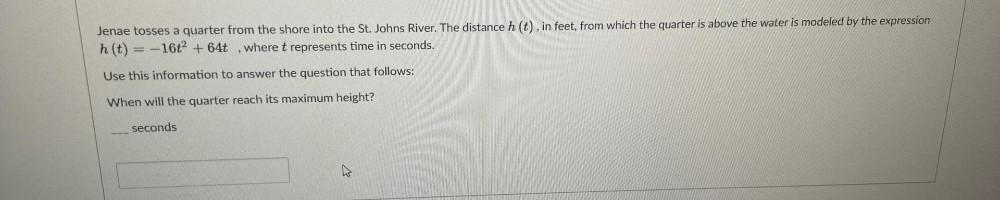Question:

# Jenae tosses a quarter from the shore into the St. Johns River. The distance h(t), in feet, from which the quarter is above the water is modeled by the expression h(t) = -16t^2+64t where t representsJenae tosses a quarter from the shore into the St. Johns River. The distance h(t), in feet, from which the quarter is above the water is modeled by the expression h(t) = -16t^2+64t where t represents time in seconds. Use this information to answer the question that follows: When will the quarter reach its maximum height? seconds